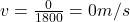## Your teacher had not exercised since the beginning of distance learning. She decided to exercise by walking in the park. The park had a rect

Question

Your teacher had not exercised since the beginning of distance learning. She decided to exercise by walking in the park. The park had a rectangular shape 200 m x 400m. She started at position A walked around the park 5 times and returned to position A in 30 min. A. Calculate the distance she walked in meters B. Calculate the displacement in meters. C. Calculate her average speed in meters/seconds D. Calculate her velocity in m/s E. Show all the unit conversations. Show all your work

in progress 0
2 months 2021-07-30T08:08:49+00:00 1 Answers 3 views 0

1. A) 6000 m

B) 0 m

C) 3.33 m/s

D) 0 m/s

Explanation:

a)

In physics, distance is a scalar quantity that represents the total length of the path covered by an object during its motion.

Being a scalar quantity, it has no direction, but only a magnitude (so, a number + its units).

The distance covered by a body can be calculated by adding together all the lengths of all the motion of the body.

In this problem, the teacher walks in the partk, starting at position A, then walking around the park 5 times and returning to the original position.

The park has a rectangular shape 200 m x 400 m, so the perimeter of the park is: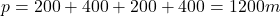The teacher covers the perimeter 5 times, so the total distance she covered is: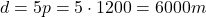B)

Displacement, instead, is a vector quantity that connects the initial position to the final position of the motion of an object.

Being a vector, it has both  a magnitude and a direction, where:

– The magnitude is equal to the length of the vector, so it corresponds to the shortest distance in a straight line between the initial and final position

– The direction goes from the initial position to the final position

In this problem:

– The teacher starts her motion at position A

– The teacher ends her motion at position A

So, the distance between the initial and final position is zero: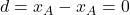And therefore, the displacement is zero.

C)

The average speed of an object is a scalar quantity telling us how fast an object is moving, regardless of its direction of motion.

Being a scalar quantity, it has only a magnitude, and no direction.

The average speed is given by the equation: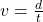where

d is the distance covered by the object

t is the time elapsed

In this problem we have:

d = 6000 m is the distance covered by the teacher

t = 30 min = 1800 s is the time elapsed

Therefore, the average speed is: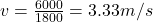D)

The average velocity of an object is a vector quantity telling us how fast an object is moving but also its direction of motion.

Being a vector, it has both a magnitude and a direction, where:

– The direction corresponds to the direction of the displacement

– The magnitude is given by the formula:where

d is the displacement of the object

t is the time elapsed

In this problem:

d = 0 is the displacement of the teacher

t = 1800 s is the time elapsed

Therefore, the average velocity is zero: